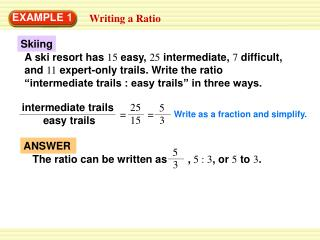Download PresentationEXAMPLE 1EXAMPLE 1 - PowerPoint PPT Presentation

Download PresentationEXAMPLE 1
An Image/Link below is provided (as is) to download presentation

Download Policy: Content on the Website is provided to you AS IS for your information and personal use and may not be sold / licensed / shared on other websites without getting consent from its author. While downloading, if for some reason you are not able to download a presentation, the publisher may have deleted the file from their server.

- - - - - - - - - - - - - - - - - - - - - - - - - - - E N D - - - - - - - - - - - - - - - - - - - - - - - - - - -
Presentation Transcript

1. Skiing A ski resort has 15 easy, 25 intermediate, 7 difficult, and 11 expert-only trails. Write the ratio “intermediate trails : easy trails” in three ways. intermediate trails 25 5 = = easy trails 15 3 ANSWER 5 The ratio can be written as , 5 : 3, or 5 to 3. 3 EXAMPLE 1 Writing a Ratio Write as a fraction and simplify.

2. Weather Lightning strikes about 100 times per second around the world. About how many times does lightning strike per minute around the world? Because 60 seconds =1 minute, 60 sec is equivalent to 1. 1 min 60 sec = 1 min 100times 100times 1sec 1sec 6000times = ANSWER 1 min Lightning strikes about 6000 times per minute around the world. EXAMPLE 2 Finding an Equivalent Rate SOLUTION Multiply by a fraction that is equivalent to 1. Simplify.

3. –24ft 24 5 = – 5sec 5 5 –4.8 = 1 ANSWER The unit rate is –4.8feet per second. Check: Round –4.8 feet per second to –5 feet per second. The product –55 = –25, which is about –24, so the answer is reasonable. EXAMPLE 3 Finding a Unit Rate Write –24 feet per 5 seconds as a unit rate. Divide numerator and denominator by 5 to get a denominator of 1 unit. Simplify.

4. expert only trails 1. easy trails = ANSWER 11 The ratio can be written as , 11 : 15, or 11 to 15. 15 15 11 2. easy trails 15 7 difficult trails = ANSWER 15 The ratio can be written as , 15 : 7, or 15 to 7. 7 for Examples 1, 2 and 3 GUIDED PRACTICE Use the information in Example 1 to write the ratio in three ways. Write as a fraction and simplify. Write as a fraction and simplify.

5. Pumping Water 3. A water pump moves 2gallons of water per second. How many gallons of water are pumped per minute? Write your answer as a rate. ANSWER 120gallons of water are pumped per minute for Examples 1, 2 and 3 GUIDED PRACTICE

6. 114 points 114 6 4. = 6 games 6 6 = ANSWER The unit rate is 19points per game. 365 people 365 5 5. = 5 5 5 months 19 73 1 1 = ANSWER The unit rate is 73people per month. for Examples 1, 2 and 3 GUIDED PRACTICE

7. 329 miles 329 10 6. = 10 gallons 10 10 32.9 = 1 ANSWER The unit rate is 32.9miles per gallon. –49 m –49 14 7. = 14 14 14 sec –3.5 m = 1sec ANSWER The unit rate is –3.5mper sec. for Examples 1, 2 and 3 GUIDED PRACTICE